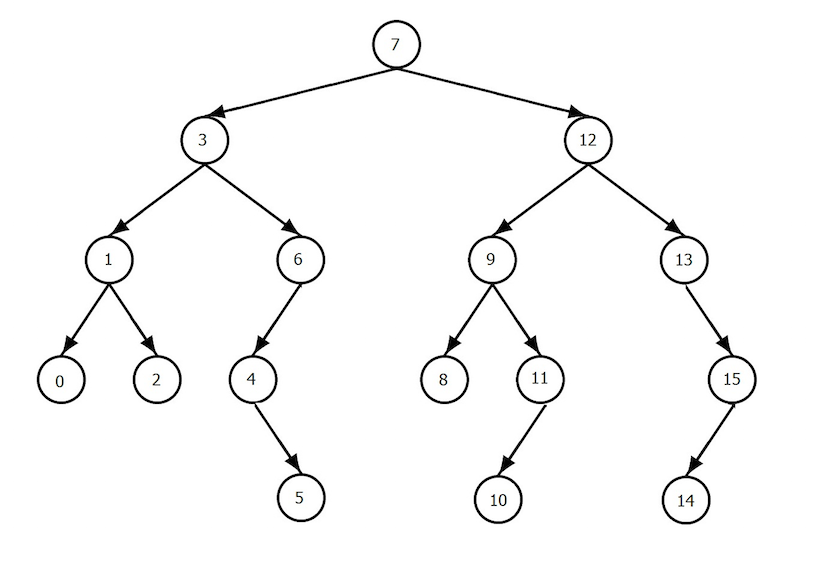I believe that the Enumerable module is the most important thing to understand if you want to go from a beginner to intermediate Rubyist. It requires you to understand two fundamental parts of Ruby: modules and blocks.

Ruby's standard library includes hashes, arrays, sets and thread-safe queues. One structure missing is a generic binary tree. Binary trees are great general purpose data structures: they aren't super fast for any operations (e.g. lookup, insert, delete) but they aren't super slow for those operations either. Databases typically implement indexes as a tree structure; every time you insert a row into a table, a node is inserted into the index's binary tree structure too. Here's what a binary tree "looks" like.Let's build a binary tree in Ruby; you will be amazed at how little code it actually takes.

Funny thing about a binary tree is that every part of the tree looks like the same: you have a node with some `data`, along with `left` and `right` pointers to the child nodes.

``````class Node
attr_accessor :data, :left, :right
def initialize(data)
@data = data
end
end

# build the first two levels of the tree pictured above
root = Node.new(7)
root.left = Node.new(3)
root.right = Node.new(12)``````

The amazing thing about `Enumerable` is this: you implement one method, `each`, and you get dozens of useful methods in return! `each` knows how to iterate through elements in your data structure and so Ruby can leverage that to implement lots of other functionality.

Remember I said that every part of a binary tree looks the same: that's a hallmark of a recursive data structure. We'll use recursion to iterate through the tree in our `each` method:

``````class Node
include Enumerable

attr_accessor :data, :left, :right
def initialize(data)
@data = data
end

def each(&block)
left.each(&block) if left
block.call(self)
right.each(&block) if right
end
end

root = Node.new(7)
root.left = Node.new(3)
root.right = Node.new(12)
root.each {|x| puts x.data } # will print "3 7 12"

puts root.inject(0) { |memo, node| memo += node.data }``````

The final trick to Enumerable is to implement a comparison operator so Ruby can compare two Nodes and tell which one is greater. This allows it to implement sorting, min and max operations. This comparison operator is commonly called the "spaceship" operator because `<=>` kinda looks like a spaceship if you squint. Note we delegate the `<=>` call to the `data` itself. We assume the tree is storing comparable data: integers, strings, or a value object which itself implements `<=>`.

``````class Node
include Enumerable

attr_accessor :data, :left, :right
def initialize(data)
@data = data
end

def each(&block)
left.each(&block) if left
block.call(self)
right.each(&block) if right
end

def <=>(other_node)
data <=> other_node.data
end
end

root = Node.new(3)
root.left = Node.new(2)
root.right = Node.new(1)
root.each {|x| puts x.data }

# just a few of the various operations Enumerable provides
puts "SUM"
puts root.inject(0) { |memo, val| memo += val.data }
puts "MAX"
puts root.max.data
puts "SORT"
puts root.sort.map(&:data)``````

This is pretty incredible and really shows off the power of Ruby: we've built a really powerful data structure in just a few lines of code. All is not wine and roses though, there's several hard parts we didn't implement (inserting a new node, deleting a node, rebalancing), I'll leave those as an exercise for the reader to steal from a StackOverflow post.

## Conclusion

Understanding and implementing Enumerable and the spaceship operator is the key to making Ruby data structures "feel" normal. In this example, the binary tree looks like any old Ruby code using an Array but is completely different under the covers.